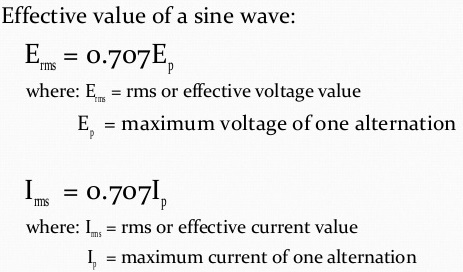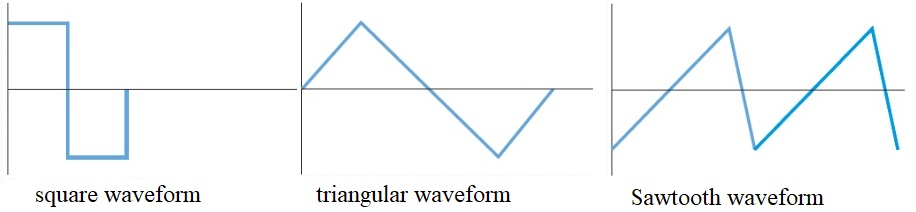# Understanding Alternating Current or AC Current

The electricity which we use at home is normally the alternating current or AC. AC current is result of alternation of the generator armature or alternator. The electricity or current flows in one direction and then reverse direction. Each cycle in AC current consists of a positive and a negative alternation.

During AC generation waveform produced is called a sine wave. If a wave completes one cycle per second then it is defined as hertz. The rms value of a sine wave is equal to 0.707 times the peak value. The relationship to understand the relation between frequency and period is:

f = 1/t

History of Alternating Current

William Stanley, Jr. designed one of the first practical devices to transfer AC power efficiently between isolated circuits. Alternating current AC power system which we see and use these days was developed rapidly after 1886, and Nikola Tesla and Carl Wilhelm Siemens has big contribution in this development.

Principle of AC Current Generation## sinusoidal waveform of alternating current

In the alternating current generation the waveform is known as sinusoidal waveform which is the most basic of the AC current wave forms. That means the other wave forms also exist which we can see at later stage. The current once produced is taken out from the alternation process using the armature of an AC generator through slip rings.

As seen from the waveform peak value of a sine wave or current is the highest point on the AC waveform having the greatest amplitude on positive side and similarly this peak value also occurs during negative alternations of the waveform. So in one waveform two peaks occur one on positive side and one on negative side. The peak-to-peak value can be determined by adding the peak values of the two alternations. Alternating current flows first in one direction and then in the other direction.

If you want to calculate the effective value of a sine wave in alternating current below is the formula for ac current:### AC Current Frequency in AC Current

Below is the formula for understanding the relationship between frequency and period of alternating current waveform:

f = 1 / t

t = 1 / f

where:

f = frequency

t = period

#### Nonsinusoidal Waveforms in AC Power

We have seen above the most common form of an ac current that is sine wave or sinusoidal waveform. There are other forms as well that include square waveform, triangular waveform and sawtooth waveform.• The most important and greatest advantage of alternating current is that AC current is transformable and DC current cannot be transformed.
• AC current can be controlled by a wide range of components like resistors, capacitors and inductors etc.
• Alternation of the current allows high-voltage electrical power to be distributed with smaller wires and lower amperage values.

### Difference between AC and DC Current (ac dc comparison)

DC current is the direct current that flows in one direction only on the other hand alternating or AC current changes direction in every cycle i.e. alternating current changes its direction 50 times in a second which we call as frequency i.e. 50 hertz per second.

AC current is used at power generation stations and ac power delivery systems and dc current is used in mostly battery and storage systems.